# Explore Biological Variability with Virtual Patients Using SimBiology Model Analyzer

This example shows how to generate and simulate virtual patients using the SimBiology Model Analyzer app. In this example, a virtual patient is represented as a single realization of model parameters. The example uses a tumor growth model  to explore the variability of some model parameters that influence the tumor growth and investigate various dosing regimens to control it. This example requires Statistics and Machine Learning Toolbox™.

### Tumor Growth Model

The model used in this example is a SimBiology® implementation of the pharmacokinetic/pharmacodynamic (PK/PD) model by Simeoni et al. It quantifies the effect of anticancer drugs on tumor growth kinetics from in vivo animal studies. The drug pharmacokinetics are described by a two-compartment model with IV bolus dosing and linear elimination (ke) from the Central compartment. Tumor growth is a biphasic process with an initial exponential growth followed by linear growth. The growth rate of the proliferating tumor cells is described by

`$\frac{{L}_{0}×{x}_{1}}{{\left[1+{\left(\frac{{L}_{0}}{{L}_{1}}×w\right)}^{\psi }\right]}^{\frac{1}{\psi }}}$`

L0, L1, and Ψ are tumor growth parameters, x1 is the weight of the proliferating tumor cells, and w is the total tumor weight. In the absence of any drugs, the tumor consists of proliferating cells only, that is, w = x1. In the presence of an anticancer agent, a fraction of the proliferating cells is transformed into nonproliferating cells. The rate of this transformation is assumed to be a function of the drug concentration in the plasma and an efficacy factor k2. The nonproliferating cells x2 go through a series of transit stages (x3 and x4) and are eventually cleared from the system. The flow-through of the transit compartments is modeled as a first-order process with the rate constant k1.

The SimBiology model makes these adjustments to the pharmacodynamics of tumor growth:

• Instead of defining the tumor weight as the sum of x1, x2, x3, and x4, the model defines the tumor weight by the reaction named Increase, null → tumor_weight, with the reaction rate $\left(\frac{2×{L}_{1}×{L}_{0}×{x}_{1}^{2}}{{L}_{1}+2×{L}_{0}×{x}_{1}}\right)×\text{tumor}\text{_}\text{weight}$. tumor_weight is the total tumor weight, x1 is the weight of the proliferating tumor cells, and L0, and L1 are tumor growth parameters .

• Similarly, the model defines the decrease in tumor weight by the reaction named Decay, tumor_weight → null, with the reaction rate k1*x4. The constant k1 is the forward rate parameter, and x4 is the last species in the series of transit reductions in tumor weight.

• ke is a function of the clearance and the volume of the central compartment: ke = Cl_Central/Central.### Description of Virtual Population

The virtual population in this example is represented by virtual patients, specified as distinct sets of patient-specific parameter values. Suppose that, based on prior knowledge or sensitivity analysis of the model (for example, see Find Important Tumor Growth Parameters with Local Sensitivity Analysis Using SimBiology Model Analyzer), the tumor growth is sensitive to these model parameters: L0, L1, w0, k1, k2, and Cl_Central. Assuming that these biological parameters follow the lognormal distribution (and must always be positive), you can generate virtual patients that represent different parameter values drawn from the joint lognormal distribution.

The lognormal distribution uses these parameters:

• mu – Mean of logarithmic values

• sigma – Standard deviation of logarithmic values

For details, see Lognormal Distribution (Statistics and Machine Learning Toolbox). In this example, sigma is assumed to be 0.01 for all the parameters. For a small sigma value, the mean of a lognormal distribution is approximately equal to the log of the model value. Hence, this example assumes that mu for each parameter is the log of the model value.

### Dosing Strategies

In this example, an anticancer drug is used to control the tumor growth. Each virtual patient receives the same amount of the drug on the same schedule. The tumor growth response is simulated for each patient. Dose amounts are then varied to find the range of dose amounts that can suppress the tumor growth of many virtual patients in the population.

Enter the following command to open the tumor growth model in SimBIology Model Analyzer. In the Browser pane of the app, the Models folder contains the Tumor Growth Model.

```openExample('simbio/VirtualPatientsSimBiologyModelAnalyzerExample') ```

### Define Joint Probability Distribution to Generate Samples to Represent Virtual Patients

The following steps show how to draw sample values from the joint probability distribution for model parameters that are sensitive to tumor growth. These sample values represent virtual patients.

Create a program to draw sample values from the joint lognormal distribution for tumor growth sensitive parameters: L0, L1, w0, k1, k2, and Cl_Central.

On the Home tab, select Program > Generate Samples. A new program opens.

In the Variants section of the program, select parameterEstimates, which contains the estimated parameter values.

Keep the Doses section as is so that no doses are selected.

In the Generate Samples step, click the plot button to disable default plot generation. You will plot the samples later on.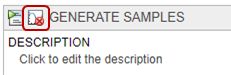In the Parameter Set section, set Type to `values from a distribution`.

Set Number Of Samples to 25.

Double-click the empty cell in the Component Name column, and enter L0.

Change Distribution for L0 to Lognormal. By definition, mu is the mean of logarithmic values. So, change mu to -0.5644, which is log(Current Value) or log(0.5687). Change sigma to 0.01. For a small sigma value, the mean of a lognormal distribution is approximately equal to log(Current Value). For details, see Lognormal Distribution (Statistics and Machine Learning Toolbox).

Double-click the empty cell in the Component Name column, and add L1. Repeat the same process to change the distribution to lognormal and set the mu and sigma values. Similarly, add w0, k1, k2, and ke. This table lists the corresponding mu values of these parameters that you can copy and paste in the software. Change sigma to 0.01 for each parameter.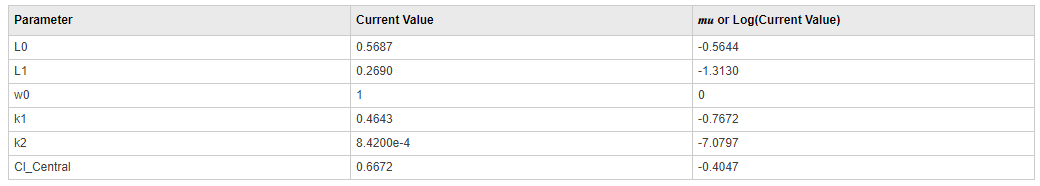For Sampling Options, use the default option: `random sampling with rank correlation matrix`, where the matrix is an identity matrix.The following figure shows the Parameter Set section with the parameters configured.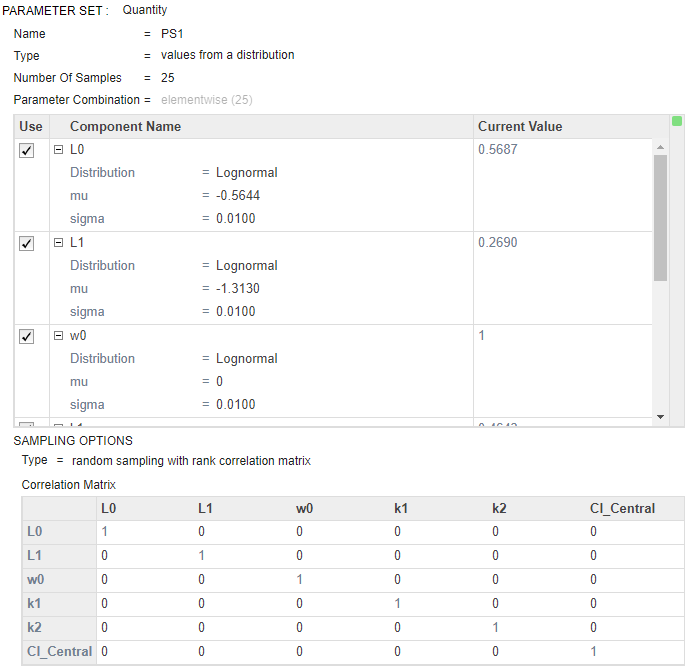(Optional) Save the project under a new name by selecting Save > Save Project As on the Home tab.

### Define Dosing Strategies

Add the dosing information as another parameter set by specifying different dose amount—specifically, six dose amounts that are equally spaced from 5 to 35 mg. Then combine the doses with the first parameter set (virtual patients) using the Cartesian combination method. This method combines every virtual patient with every dose amount to generate a total of 150 iterations (or simulation scenarios). For details, see Combine Simulation Scenarios in SimBiology.

At the bottom of the Generate Samples step, click Add parameter set to scan. Another parameter set (PS2) appears.

Double-click the empty cell in the Component Name column, and type interval. A list of choices appears. Select interval_dose.Amount.

Set Type to `Individual Values` and set Values to [5:6:35].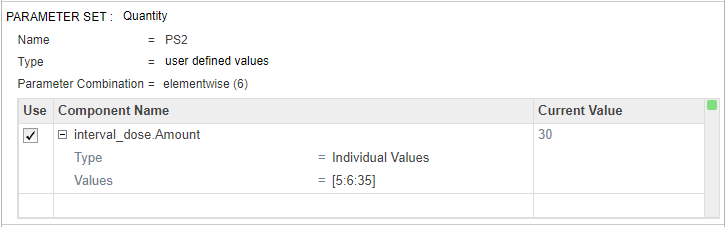Near the top of the Generate Samples step, under Parameter Set Combinations, ensure that the Combination type is set to cartesian to combine PS1 and PS2.### Generate and Visualize Parameter and Dose Combinations

Once you have defined the joint lognormal distribution for model parameters, range of dose amounts, and the combination method, you can run the step to generate the different parameters and dose combinations.

Click the Run this program step button to generate samples.Tip: You can run every program execution step separately. An execution step includes the run button next to the name the step. Running an individual step is especially helpful if the program contains multiple steps and you want to see the intermediate results from a particular step. By doing so, you can make adjustments as needed before running the next step or the whole program. To run the whole program, click the Run button on the Home tab.

By default, the app stores the generated samples in the LastRun folder of the program.

Visualize the generated samples. In the Browser pane on the left, expand the Program1 folder. Expand the LastRun folder, and then click samples. In the Plot section on the Home tab, click Plot Matrix. The plot shows the distribution of each parameter varied around its model value, except the dose amounts, which appear in a uniform range. Note that your plot might vary from the plot shown here due to randomness.Note: You can enable default plot generation to display the plot automatically every time you run the step. To do so, click the plot button at the top of the Generate Samples step. Keep in mind that when the program step has a lot of samples, plotting all the samples can be time consuming.

Display the generated virtual patients and dose combinations numerically in a tabular format. On the Home tab, click New Datasheet. From the LastRun folder of the program, drag samples into the new datasheet. Each row of the table represents a simulation scenario with different model parameter values and dose amounts.### Perform Monte Carlo Simulations

Once you have the samples ready, you can simulate the model to explore the tumor growth of virtual patients receiving different dose amounts.

Go back to the program by clicking the Program1 tab.

Click the (+) icon at the upper left and click Simulation.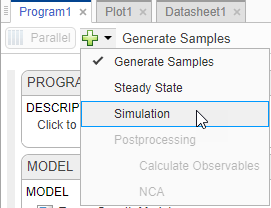In the Model setup step, in the Variants section, make sure that parameterEstimates is selected. Also make sure that no doses are selected in the Doses section. In the States To Log section, click the option for viewing the states, and then clear all check boxes except [Tumor Growth Model].tumor_weight.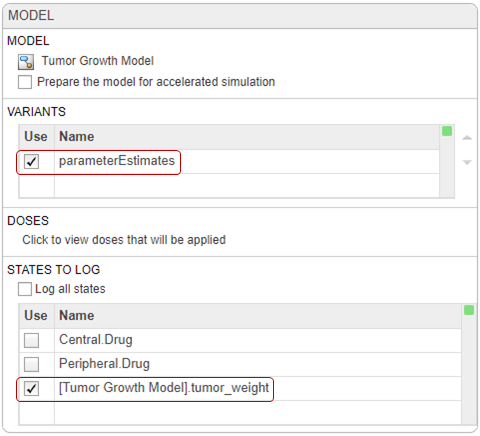At the top of the Simulation step (scroll down to see this step), click the Run this program step button to simulate the model.

The app simulates the generated scenarios from the Generate Samples step that you ran previously. You do not need to rerun that step. Once the simulation finishes, the app saves the simulation results in the LastRun folder and opens Plot2, showing a percentile plot of the simulation result.

In the Property Editor pane, under Style, click Time. Each line in the plot represents the tumor weight profile of each simulation scenario.The plot shows that the tumor weight profiles appear in groups, corresponding to different dose amounts. To better visualize this observation, you can slice the data by dose amounts. To do so, you can use the Slice Data table, which contains a summary of slicing variables that are currently being used in the plot and their corresponding plot styles.

Tip: Plots are backed by data that are currently present in the app workspace. Plots are not snapshots. When the data (either experimental data or simulation results) is removed or changed, the plots are also updated according to the changes in the underlying data.

In the Visual Channels table of Property Editor, double-click L0 and select interval_dose Amount.Tip: You can slice data using different slicing variables. Each slicing variable appears in the plot with a different visual style (or channel) such as color, line style, and axes position. Slicing variables can represent attributes of data, such as responses or scenarios (that is, groups or simulation runs). Slicing variables can also be covariates or parameter values associated with a scenario or group. By default, the app provides slicing variables for different response variables and different scenarios in the plotted data. You can add other visual styles (or channels) for sets of responses and associated parameter or covariate variables.

Double-click the empty cell in the Style column and select Grid.The app updates Plot2 to show each dose amount on its own axes.The plot shows that different dose amounts play critical roles in the tumor growth of virtual patients. From this plot, you can obtain some initial insights into the optimal dose amounts and dose scheduling. For instance, suppose that the target tumor weight to reach is 0.5 grams. The simulation results indicates that a dose amount of 23 milligrams or larger can achieve that goal (you can fine-tune the range of dose amounts further by tweaking it in the Generate Samples step of the program). You can use this information in combination with existing drug toxicity information (not discussed in this example) to get a dosing regimen that satisfies both efficacy and safety requirements, for instance.

### Postprocess Simulation Data

You can also postprocess the simulation results to look at the correlation between dose amounts and the tumor weight in another way.

Go back to the program by clicking the Program1 tab. Click the (+) icon near the top of the program and select Calculate Observables under Postprocessing.A Postprocessing: Calculate Observables step appears below the Simulation step.

Double-click the first cell in the Name column. Enter `stat1`.

In the Expression column, enter the following expression: `min(vertcat(nan,tumor_weight(time>=7)))`. The expression returns the minimum tumor weight after the first dose applied at day 7 from each simulation. Note: Anytime you add an expression to the Observables table in the step, the expression is automatically added as an observable object to the corresponding model.

In the Units column, enter gram.

Rerun the simulation step by clicking the run button at the top of the step. The app simulates and evaluates the stat1 expression for each simulation scenario or iteration, and generates the following plot. The x-axis represents the parameters and the y-axis represents the minimum tumor weight. Each dot represents a simulation scenario. The plot also shows that there is no correlation between the tumor size and the values of various model parameters, except for the dose amounts (the rightmost subplot).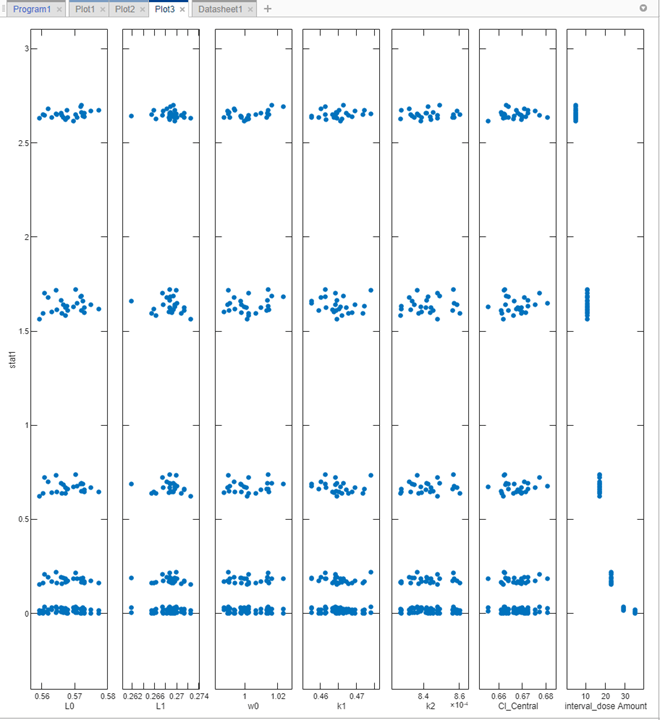Show only the dose amount subplot. In the Plot Settings, clear all check boxes in the Parameters table, except interval_dose Amount.

In the Grid section, select both to show the grids for both the x- and y-axis.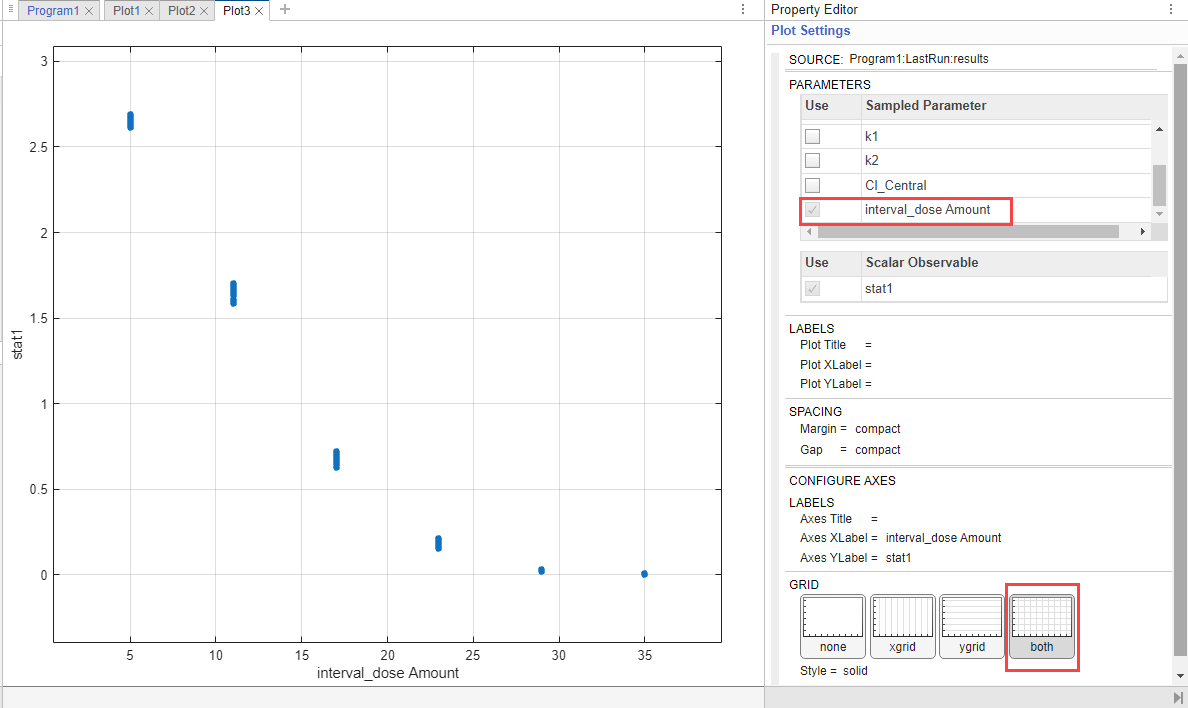The plot confirms that higher dose amounts control the tumor growth better. These initial simulation results indicate that the dose amounts of 23 mg or larger could reach the hypothetical tumor weight threshold of 0.5 grams or lower. You can further adjust the range of dose amounts in the Generate Samples step.

Tip: To interactively explore the plotted data, export the plot to a separate figure window by selecting Export Plot from the context (right-click) menu of the plot.

This example shows you how to generate samples to represent virtual patients and perform Monte Carlo simulations to explore the model response on tumor growth under different dose amounts. The simulation results indicate a range for dose amounts and dose schedules that controls the tumor growth for various virtual patients. You could further adjust the dosing regimens so that the doses stay within some known efficacy and toxicity thresholds. For a similar analysis, see the example Scan Dosing Regimens Using SimBiology Model Analyzer App.

 Simeoni, Monica, Paolo Magni, Cristiano Cammia, Giuseppe De Nicolao, Valter Croci, Enrico Pesenti, Massimiliano Germani, Italo Poggesi, and Maurizio Rocchetti. “Predictive Pharmacokinetic-Pharmacodynamic Modeling of Tumor Growth Kinetics in Xenograft Models after Administration of Anticancer Agents.” Cancer Research 64, no. 3 (February 1, 2004): 1094–1101.

 Koch, Gilbert, Antje Walz, Gezim Lahu, and Johannes Schropp. “Modeling of Tumor Growth and Anticancer Effects of Combination Therapy.” Journal of Pharmacokinetics and Pharmacodynamics 36, no. 2 (April 2009): 179–97.